Proof - Using properties of sets

Chapter 1 Class 11 Sets
Concept wise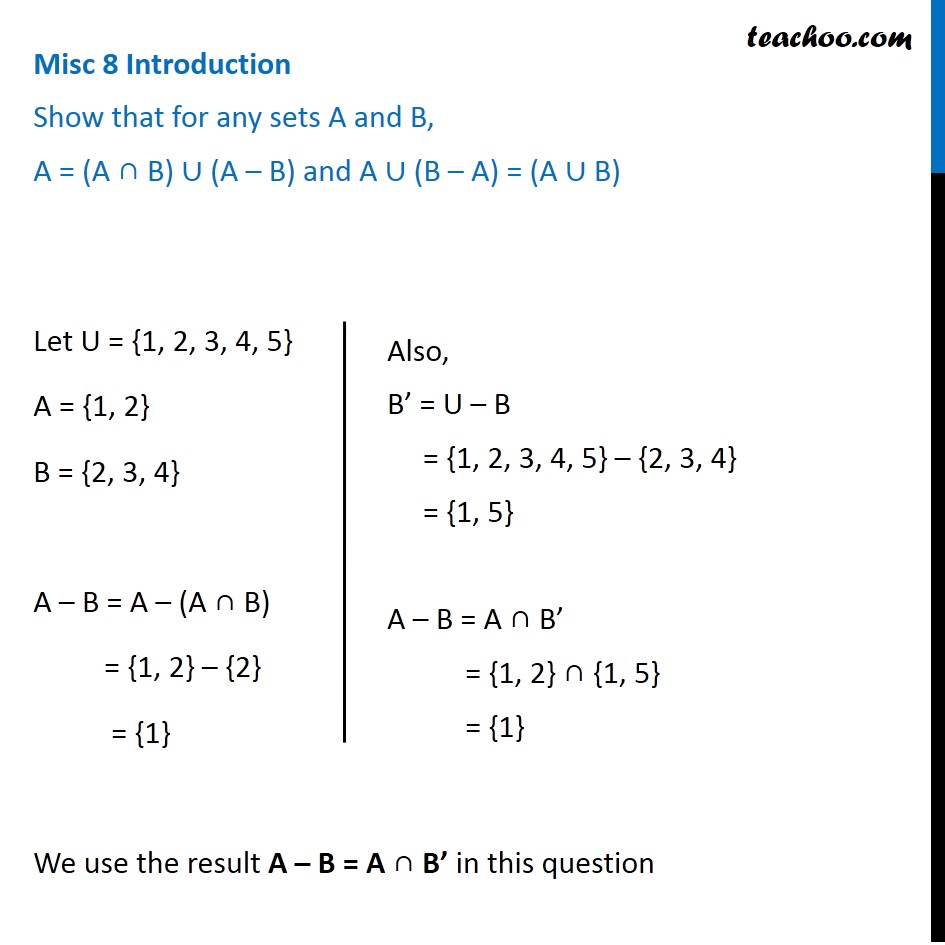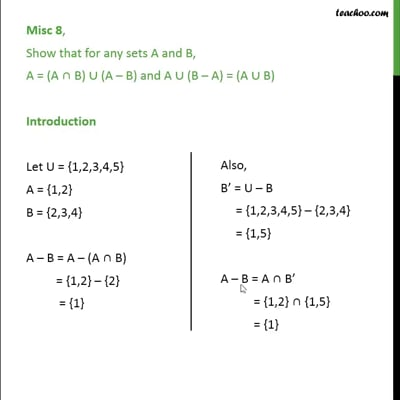This video is only available for Teachoo black users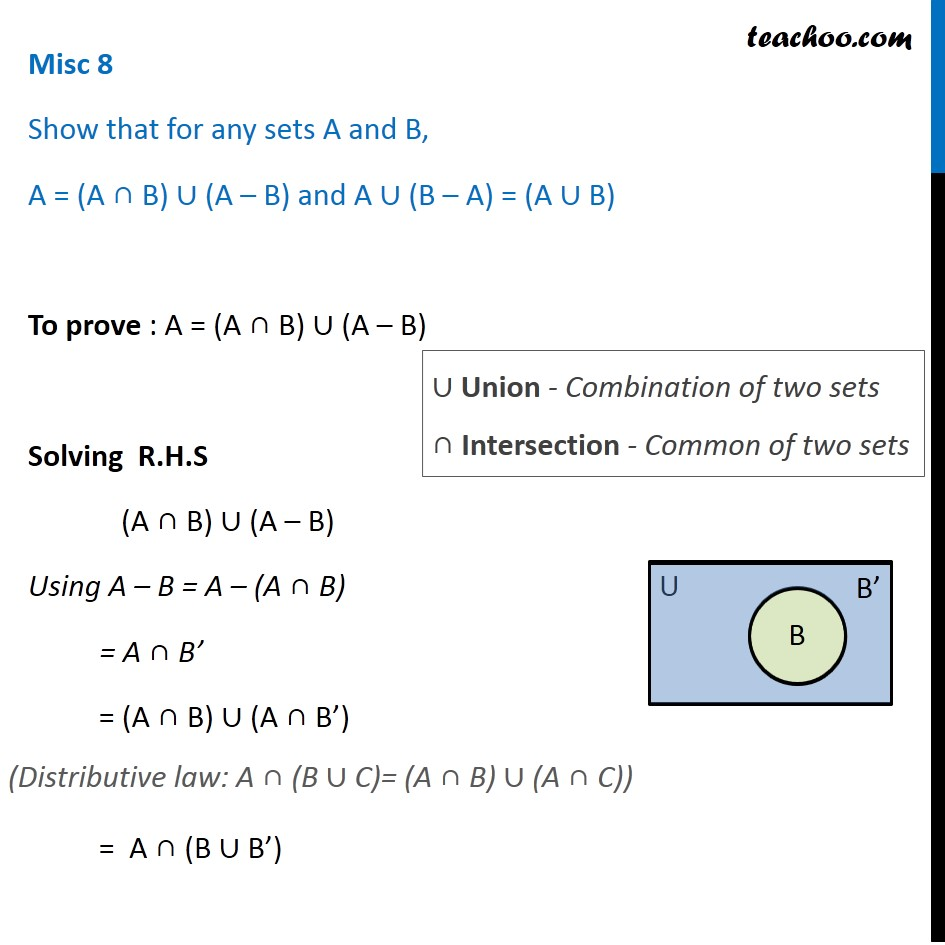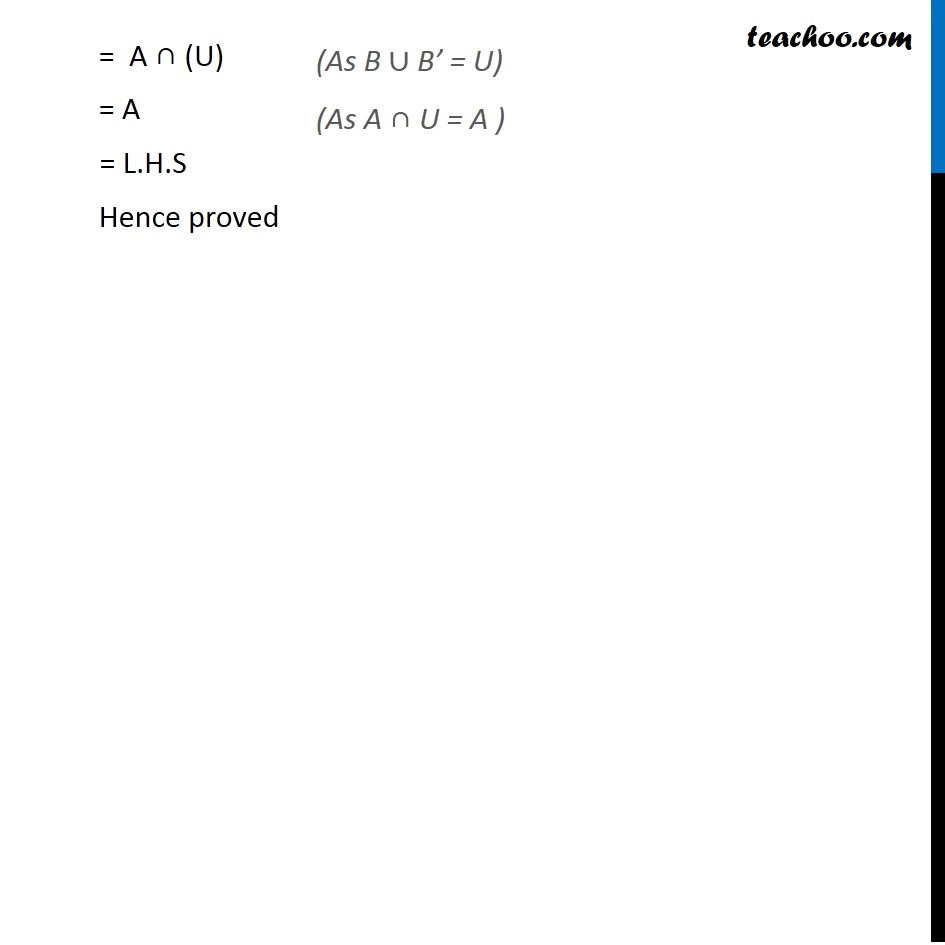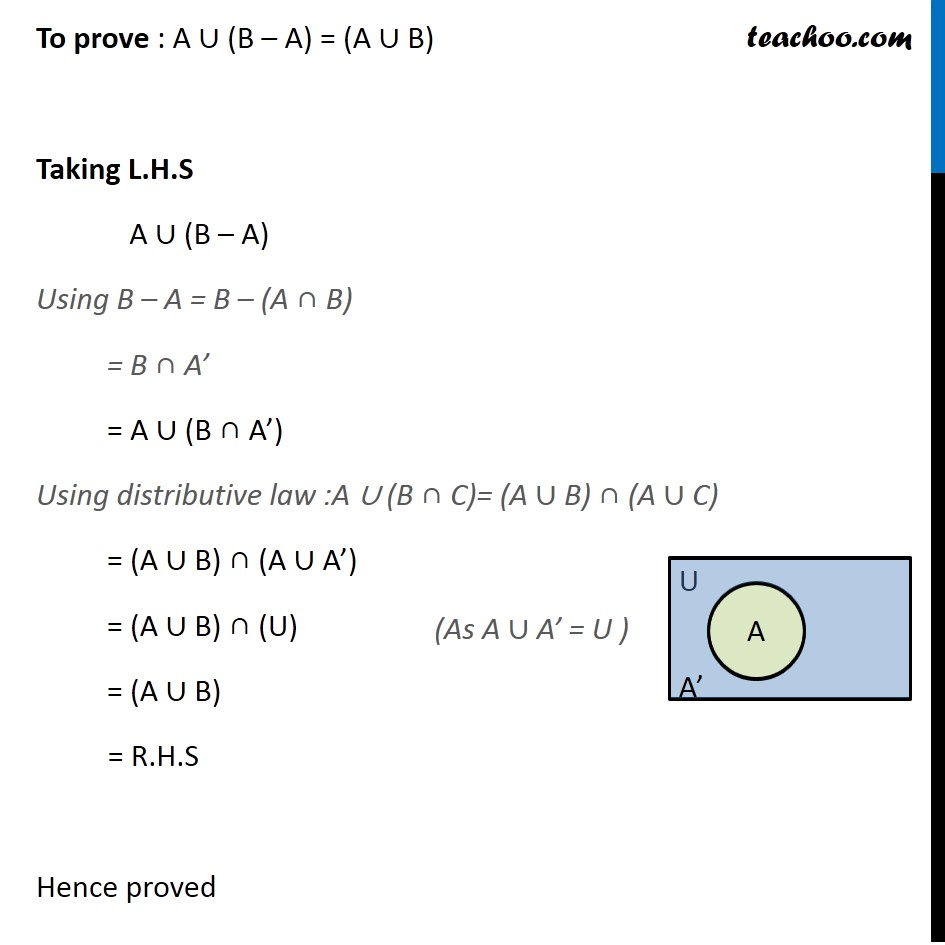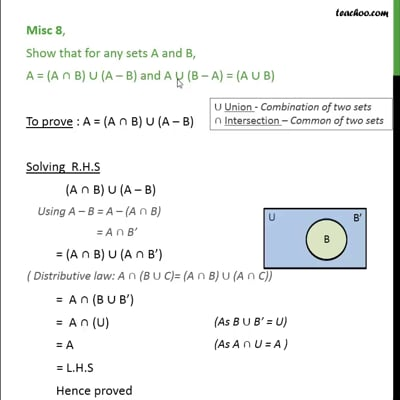This video is only available for Teachoo black users

Solve all your doubts with Teachoo Black (new monthly pack available now!)

### Transcript

Misc 8 Introduction Show that for any sets A and B, A = (A ∩ B) ∪ (A – B) and A ∪ (B – A) = (A ∪ B) Let U = {1, 2, 3, 4, 5} A = {1, 2} B = {2, 3, 4} A – B = A – (A ∩ B) = {1, 2} – {2} = {1} We use the result A – B = A ∩ B’ in this question Also, B’ = U – B = {1, 2, 3, 4, 5} – {2, 3, 4} = {1, 5} A – B = A ∩ B’ = {1, 2} ∩ {1, 5} = {1} Misc 8 Show that for any sets A and B, A = (A ∩ B) ∪ (A – B) and A ∪ (B – A) = (A ∪ B) To prove : A = (A ∩ B) ∪ (A – B) Solving R.H.S (A ∩ B) ∪ (A – B) Using A – B = A – (A ∩ B) = A ∩ B’ = (A ∩ B) ∪ (A ∩ B’) = A ∩ (B ∪ B’) ∪ Union - Combination of two sets ∩ Intersection - Common of two sets (Distributive law: A ∩ (B ∪ C)= (A ∩ B) ∪ (A ∩ C)) = A ∩ (U) = A = L.H.S Hence proved (As B ∪ B’ = U) (As A ∩ U = A ) To prove : A ∪ (B – A) = (A ∪ B) Taking L.H.S A ∪ (B – A) Using B – A = B – (A ∩ B) = B ∩ A’ = A ∪ (B ∩ A’) Using distributive law :A ∪ (B ∩ C)= (A ∪ B) ∩ (A ∪ C) = (A ∪ B) ∩ (A ∪ A’) = (A ∪ B) ∩ (U) = (A ∪ B) = R.H.S Hence proved (As A ∪ A’ = U )